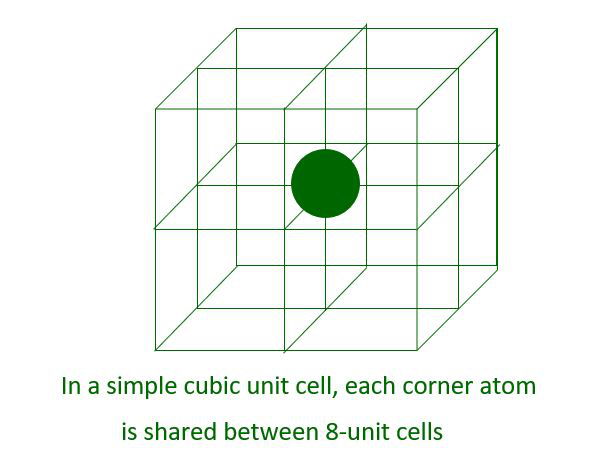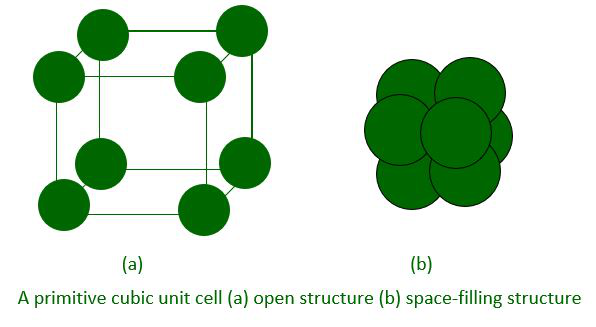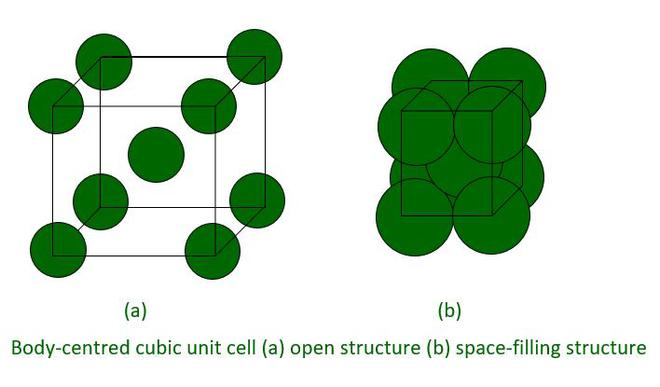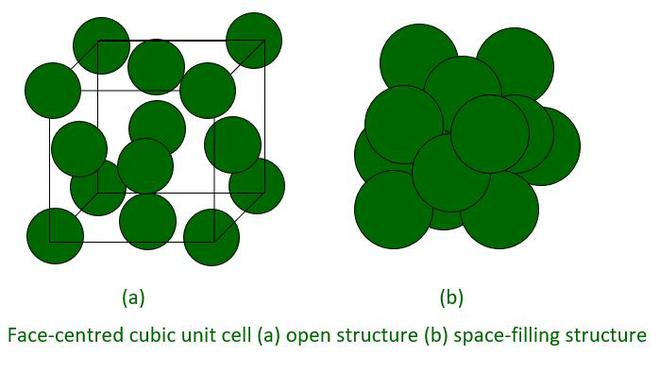Open In App
Related Articles
• CBSE Class 12 Chemistry Notes

# Calculate the Number of Particles per unit cell of a Cubic Crystal System

We are mostly surrounded by solids, which we use more frequently than liquids and gases. We require solids with a wide range of properties for various applications. These properties are determined by the nature of the constituent particles and the binding forces that exist between them. As a result, studying the structure of solids is critical.

Solids are classified into two types: crystalline and amorphous. This article will teach us more about crystalline solids. Crystalline solids are distinguished by a regular and repeating pattern of constituent particles. The three-dimensional arrangement of constituent particles in a crystal is known as crystal lattice if it is represented diagrammatically, with each particle depicted as a point.

The smallest portion of a crystal lattice that, when repeated in different directions, produces the entire lattice. Unit cells are three types:

1. Primitive Unit Cells
2. Body-centered Unit Cells
3. Face-centered Unit Cells

### Number of Particles in Unit Cells

We know that any crystal lattice is composed of a large number of unit cells and that each lattice point is occupied by one constituent particle (atom, molecule or ion). Let us now determine which portion of each article belongs to which unit cell. For the sake of simplicity, we will consider three types of cubic unit cells and assume that the constituent particle is an atom.

• Primitive Cubic Unit CellIn Fig.(a), each small sphere represents only the centre of the particle occupying that position, not its actual size. Such structures are referred to as open structures. In open structures, particle arrangement is easier to follow. Figure (b) shows a space-filling representation of a unit cell with actual particle size.Since each cubic unit cell has 8 atoms on its corners, the total number of atoms in one unit cell is-

Number of atoms in simple cubic unit cell = (Number of  corners)×(Part of atom in each unit cell)

= (8)×(1/8)

= 1 atom.

• Body-Centered Cubic Unit Cell

A body-centered cubic (bcc) unit cell has an atom at each of its four corners, as well as one atom in the centre. It can be seen that the atom at the centre of the body belongs entirely to the unit cell in which it is found.Thus in a body-centered cubic (bcc) unit cell atoms are present at all the corners and at the body centre of the unit cell.

Total number of atoms per unit cell = Atom at corners + Atom at body center

= (8 corners × 1/8 per corner atom) + (1 atom at body centre)

= 8 × 1/8 + 1

= 1 + 1

= 2 atoms.

Face-centered Cubic Unit Cell

A face-centered cubic (fcc) unit cell has atoms at all of the cube’s corners and in the centre of all of its faces. Each atom in the centre of the face is shared by two adjacent unit cells, and only half of each atom belongs to a unit cell.Thus, a face-centered cubic (fcc) unit cell has atoms at all the corners and all the faces of the unit cell.

Total number of atoms per unit cell = Atoms at corners + Atoms at the face of the unit cell

= (8 corners × 1/8 per corner atom) + ( 6 faces × 1/2 per face atom)

= 8 × 1/8 + 6 × 1/2

= 1 + 3

=  4  atoms.

### Solved Questions

Question 1: Solids are rigid why?

Solids are rigid because the constituent particles of solid has fixed position and there is no relative motion between the particles.

Question 2: What are lattice point and their significance?

The position of a specific constituent in the crystal lattice is denoted by the lattice point. This lattice point can be an atom, an ion, or a molecule. The shape of a crystalline solid is determined by the arrangement of the lattice points in space.

Question 3: Give an example of crystalline solids?

Example of crystalline solids are salt (Sodium Chloride), Quartz, Diamond, etc.

Question 4: How many atoms are present in the face-centered cubic unit cell at the following position-

1. at corners,
2. at faces and
3. at centre

In face-centered cubic unit cell we know that atoms are present at all the corners and at all the face of unit cell.

1. Number of atom at corners = 8 corner × 1/8 per corner atom = 8 × 1/8  = 1 atom at corner
2. Number of atom at face  =  6 face × 1/2 per face atom  =  6×1/2  = 3 atoms at face  of unit cell
3. Number of atom at center = 0 (because in face-centered cubic unit cell no atom is present at center).

Question 5: What are the parameters that define a unit cell?